zoukankan      html  css  js  c++  java
• # Day01 基础篇（二）Python基础语法快速梳理

一、Python基础语法

1、输入输出语句

input为python标准的输入函数

printf为python标准的输出函数

%name和%sum代表变量的数值，因为两者其一指代字符串，其二指代数字，所以采用%s和%d代替

```name = input("What do you want to say:")
sum = 100+200
print("I want to say:%s" %name)
print("100+200=%d" %sum)```
```What do you want to say:Hello World!
I want to say:Hello World!
100+200=300```

2、if...else...语句

if...else...是经典的判断语句，注意要在条件表达式后面加上:

代码缩进在Python中是一种语法，缩进不一致有可能会导致错误

```score = 98
if score>=90:
print("优秀")
elif score>=80 and score<90:
print("良好")
elif score>=60 and score<80:
print("合格")
else:
print("不合格")```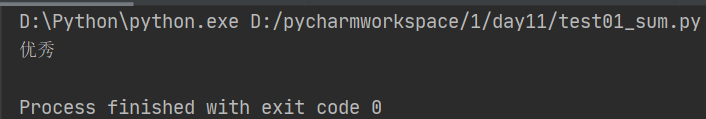3、for...in

for 循环是一种迭代循环机制，迭代即重复相同的逻辑操作。如果规定循环的次数，我们可以使用 range 函数，它在 for 循环中比较常用。range(11) 代表从 0 到 10，不包括 11，也相当于 range(0,11)，range 里面还可以增加步长，比如 range(1,11,2) 代表的是[1,3,5,7,9]。

```sum = 0
for i in range(11):
sum += i
print(sum)```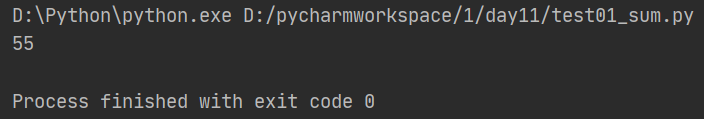4、while

while 循环是条件循环，在 while 循环中对于变量的计算方式更加灵活。因此 while 循环适合循环次数不确定的循环，而 for 循环的条件相对确定，适合固定次数的循环。

```sum = 0
num = 1
while num<11:
sum+=num
num = num+1
print(sum)```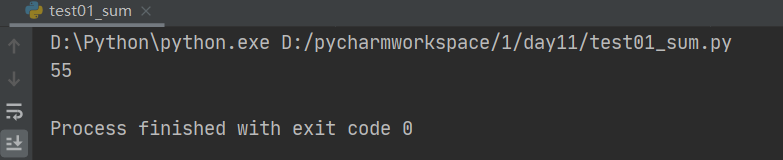5、列表

列表相当于数组，具有增删改查功能，len()可以获取列表中元素的个数，append()可以在尾部添加元素，使用insert()在列表指定位置插入元素，使用pop()删除尾部元素。

```lists = ['a','b','c']
lists.append('d')
print(lists)
print(len(lists))
lists.insert(0,'mm')
lists.pop()
print(lists)```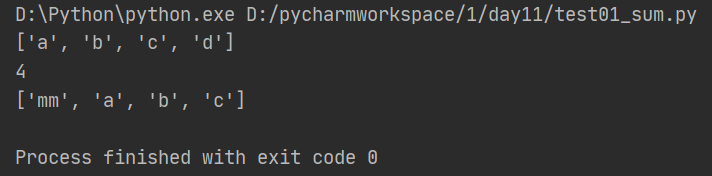6、元组

元组和列表非常相似，但是元组一旦初始化不能修改，即不能增删改，可以查找。

```tuples = ('tupleA','tupleB')
print(tuples)```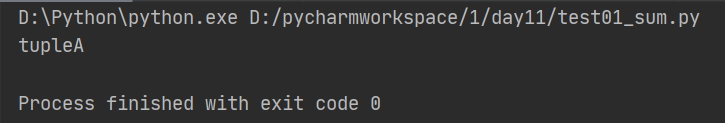7、字典

字典其实就是{key:value,...}，多次对同一key放入value，后面的值会把前面的值冲掉，同样字典也具有增删改查功能，增加字典元素相当于赋值，例如score['zhaoyun'] = 98。删除一个元素使用pop(),查询一个元素使用get(),如果查询不存在我们可以给定默认值，比如score.get('yase',99)，即不存在就将值设为99

```#定义一个字典
score = {'guanyu':95,'zhangfei':96}
# 添加一个元素
score['zhaoyun'] = 98
print(score)
# 删除一个元素
score.pop('zhangfei')
# 查看key是否存在
print('guanyu' in score)
# 查看一个key对应的值
print(score.get('guanyu'))
print(score.get('yase',99))```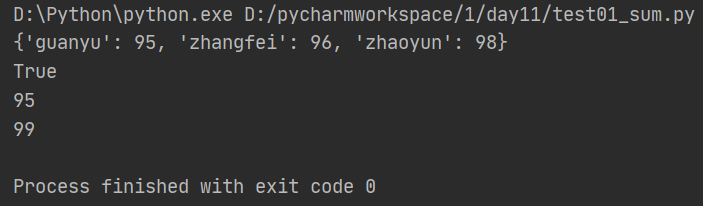8、集合

```s = set(['a','b','c'])
s.remove('b')
print(s)
print('c' in s)```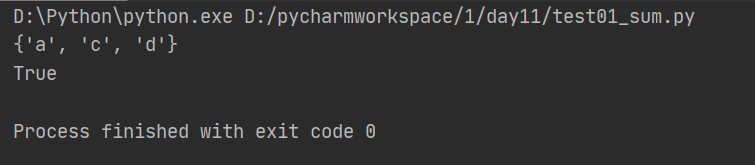9、引用模块/包

import的本质是路径搜索，import可以引用模块module或者包package

module的引用实际上是引用了一个.py文件，针对package可以使用from...import...的方式，实际上是从一个目录中引用模块，这时目录结构中必须带有一个_init_.py文件

```# 导入一个模块
import model_name
# 导入多个模块
import module_name1,module_name2
# 导入包中指定模块
from package_name import moudule_name
# 导入包中所有模块
from package_name import *```

10、函数

函数代码块以def关键词开头，后接函数标识符名称和圆括号，在圆括号内传入参数，通过return进行函数结果的返回。

```def addone(score):
return score +1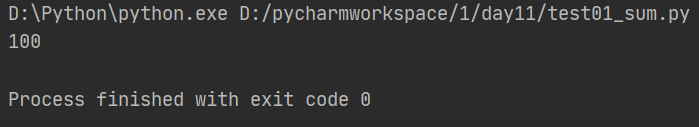11、实战练习题目

（1）实现A+B

输入格式：有一系列的整数对A和B，以空格分开

输出格式：对于每个整数对A和B，需要给出A和B的和

输入输出样例：

```INPUT
1 5
OUTPUT
6```

标准答案：

```while True:
try:
line = input()
a = line.split()
print int(a)+int(a)
except:
break```

(2)在python中引入scikit-learn库应当如何引用

```#安装scikit-learn
sudo pip intall -U scikit-learn
#导入scikit-learn 库
from sklearn import *```

(3)求1+3+5+7+…+99的求和，用Python该如何写？

```sum = 0
for i in range(1,100,2):
sum+=i
print("1+3+5+7+...+99的求和为%d"%sum)```
• 相关阅读:
【转】数学题目大集合
hdu3534，个人认为很经典的树形dp
GYM
HDU
POJ
POJ
POJ
set的经典应用
天梯赛训练1 7-9 集合相似度
天梯赛训练1 7-8 查验身份证
• 原文地址：https://www.cnblogs.com/MurasameLory-chenyulong/p/14278762.html